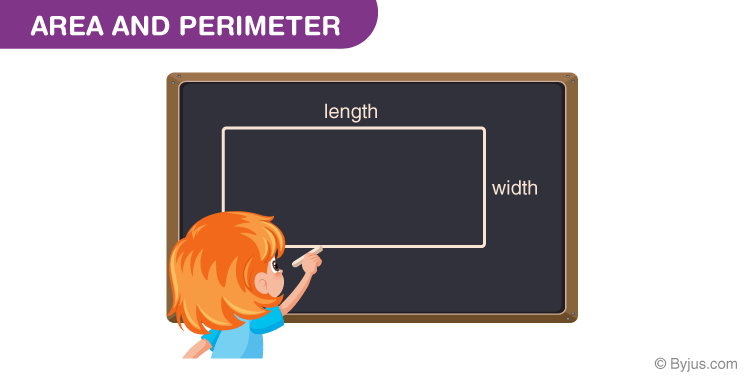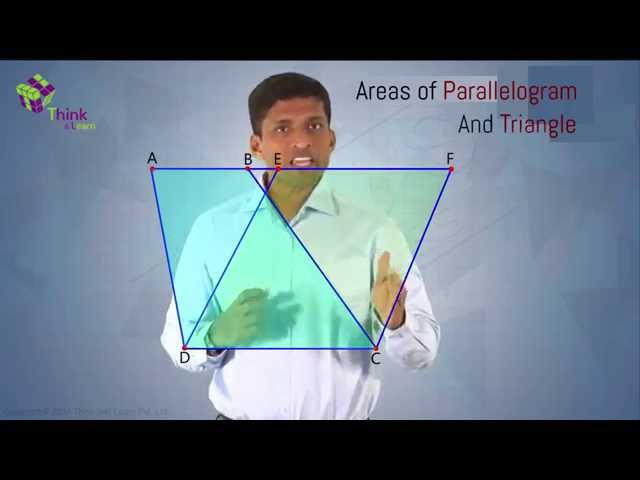# Area and Perimeter Formula

Area and Perimeter Formula are the two major formulas for any given two-dimensional shape in Mathematics. In geometry, you will come across many shapes such as circle, triangle, square, pentagon, octagon, etc. In real life as well, you will come across different types of objects having different shapes and sizes, which occupy some space in a place and their outline distance specifies the total length of the object.

All the shapes have their own properties, based on their structure, sides and angles. The two main features are the area and perimeter. For example, the amount of paint required to paint a wall of rectangular shape is calculated by its area and to put the boundaries of the square field we have to calculate its perimeter to know the total length of the field.

Every geometrical shape has its area and perimeter. There are different formulas for area and perimeter of every shape as it has a different measurement. So, let us learn the area and perimeter formulas for all shapes, here.

## Area Formula

The area is the measurement of space enclosed by a closed geometric figure. Like the perimeter formula, there is also a set of area formula for polygons that can be represented using algebraic expressions.

For example, if you want to know the area of a square box with side 40 cm, you will use the formula:

Area of Square = a2, where a is the side of the square.

Similarly, the area of a triangle can also be found using its Area formula (1/2 × b ×h).

## Perimeter Formula

A Perimeter is the length of the boundary of a closed geometric figure. Algebraic expressions can be used to represent the perimeter formula for the regular polygons. Say that the length of each side of a regular polygon is l. The perimeter of shapes formula for each of the polygons can be given using the same variable l.

Example: To find the perimeter of a rectangular box, with length as 6 cm and Breadth as 4 cm,  we need to use the formula,

Perimeter of a Rectangle = 2 (L+B) = 2 (6 cm + 4 cm) = 2 × 10 cm = 20 cm.### Area and Perimeter Formula Chart

Area Formulas for different geometrical figures:

 Figures Area Formula Variables Area of Rectangle Area = l × w l =  length w  = width Area of Square Area  = a2 a = sides of the square Area of a Triangle Area = 1/2 b×h b = base h = height Area of a Circle Area = πr2 r = radius of the circle Area of a Trapezoid Area = 1/2 (a + b)h a =base 1 b = base 2 h = vertical height Area of Ellipse Area = πab a = radius of the major axis b = radius of the minor axis

Perimeter Formulas for different geometrical figures:

 Geometric Shape Perimeter Formula Metrics Parallelogram 2(Base + Height) Triangle a + b + c a, b and c being the side lengths Rectangle 2(Length + Width) Square 4a a =Length of a side Trapezoid a +b+c+d a, b, c, d being the sides of the trapezoid Kite 2a + 2b a = Length of the first pair of equal sides b = Length of the second pair of equal sides Rhombus 4 x a a  = Length of a side Hexagon 6 x a a  = Length of a side

### Area and Perimeter of Special Triangle

An Equilateral triangle is a special triangle having all the three sides equal. To find its perimeter and area we need to know all the three sides of it.

Equilateral Triangle

• The perimeter of an equilateral triangle = 3 x length of a side = 3l.
• Area of an equilateral triangle = √3 / 4 a2

### Solved Examples

Let us see some of the examples using Area and perimeter formulas:

Example 1:  Find the perimeter of a rectangular box, with length as 6 cm and breadth as 4 cm.

Solution:  Use the formula,

Perimeter of a Rectangle = 2 (L+B) = 2 ( 6 cm + 4 cm) = 2 × 10 cm = 20 cm.

Example 2: How to find the area and perimeter of a square? Find the perimeter of a square if the area is 36 cm2.

Solution: A square is a shape with all the four sides equal in length. These four sides are also parallel to each other. They also make an angle of 90° with each other. To find the area and perimeter of the square, we need to know the measurement of one side of the square.

Area of a square = (Side)2, and

Perimeter of a square = 4(Side)

Given: Area is 36 cm2

(Side)2  = 36

Or Side = 6 (Ignored negative value as length cannot be negative)

Again, using the perimeter formula, we have

Perimeter = 4(Side) = 4 x 6 = 24

So, 24 cm is the perimeter of a square.

Area and Perimeter is a very important topic in Maths and students are advised to go through the list of formulas listed above before working on the problems for better understanding and preparation.## Practice Questions

1. Find the area of a rhombus of perimeter 60 cm and one of its diagonals is 24 cm.
2. Find the area and perimeter of an equilateral triangle of side 8 cm.
3. A circular track runs around a circular park. If the difference between the circumference of the track and the park is 66 m, then find the width of the track.

Download BYJU’S-The Learning App and get personalised videos for all the mathematical concepts from Class 1 to 12.

## Frequently Asked Questions on Area and Perimeter Formula

Q1

### What is meant by the area and perimeter of any shape?

Area means the region enclosed by any closed figure and perimeter means the length of the boundary of the shape.

Q2

### What is the area and perimeter of an equilateral triangle?

Area of equilateral triangle = √3/4 a2 and perimeter of an equilateral triangle = 3a where a is the length of the side of the equilateral triangle.

Q3

### What is the area and perimeter of an isosceles triangle?

Area of isosceles triangle = b/4[4a2 – b2]1/2 and perimeter of an isosceles triangle = 2a + b where a is the length of the equal sides and b is the length of the unequal side.

Q4

### What is the area of a trapezium?

Area of a trapezium = ½ h(a + b) where h = height and a, b are length of the parallel sides.